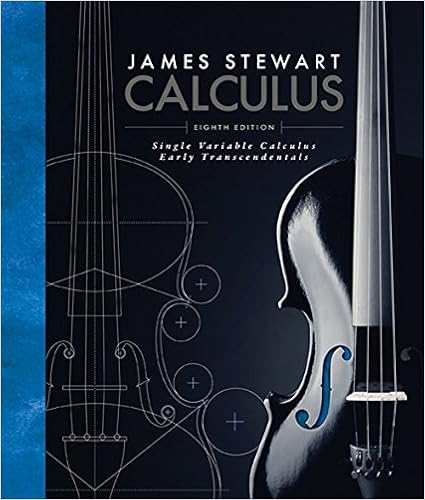# Differentiate the function

• Homework Help
• 6
• 50% (2) 1 out of 2 people found this document helpful

This preview shows page 1 - 6 out of 6 pages.

##### We have textbook solutions for you!
The document you are viewing contains questions related to this textbook.The document you are viewing contains questions related to this textbook.
Chapter 3 / Exercise 25
Single Variable Calculus: Early Transcendentals
StewartExpert Verified
Name: Tanya Snyder College ID: 0397884 Thomas Edison State University Calculus I (MAT-231) Section no.: GS005 Semester and year: October 2018 Written Assignment 5 Answer all assigned exercises, and show all work. Each exercise is worth 5 points. 1. Differentiate the function.
2. Differentiate the function.
3. Differentiate the function.
##### We have textbook solutions for you!
The document you are viewing contains questions related to this textbook.The document you are viewing contains questions related to this textbook.
Chapter 3 / Exercise 25
Single Variable Calculus: Early Transcendentals
StewartExpert Verified
4. Compute the indicated derivative. 4
5. Use the given position function to find the velocity and acceleration functions.
6. Find an equation of the tangent line to 𝑦𝑦 = 𝑓𝑓 ( 𝑥𝑥 ) at 𝑥𝑥 = 𝑎𝑎 . = 2
7. Find the derivative. 𝑥𝑥
WA 5, p. 3 8. Find the derivative. 𝑥𝑥 2 2 𝑥𝑥 𝑓𝑓 ( 𝑥𝑥 ) = 𝑥𝑥 2 + 5 9. Find an equation of the tangent line to the graph of 𝑦𝑦 = 𝑓𝑓 ( 𝑥𝑥 ) at 𝑥𝑥 = 𝑓𝑓 ( 𝑥𝑥 ) = ( 𝑥𝑥 3 + 𝑥𝑥 + 1)(3 𝑥𝑥 2 + 2 𝑥𝑥 − 1 ), 𝑎𝑎 = 1 10. Differentiate each function. (a) 𝑓𝑓 ( 𝑡𝑡 ) = ( 𝑡𝑡 4 + 2)√𝑡𝑡 2 𝑥𝑥 𝑎𝑎 . + 1 4 (b) 𝑓𝑓 ( 𝑡𝑡 ) = √𝑡𝑡 ( 𝑡𝑡 3 + 3)
11. Differentiate each function.
WA 5, p. 4
•••